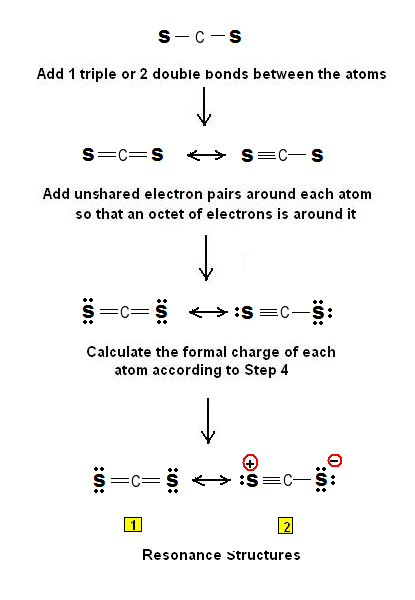Simple Method for writing Lewis Structures for Carbon Disufide CS2 | Chemistry Net

# Simple Method for writing Lewis Structures for Carbon Disufide CS2

Simple Method for writing Lewis Structures for Carbon Disulfide CS2

# Simple Method for writing Lewis Structures for Carbon Disulfide CS2

A simple procedure for writing Lewis Dot Structures was given in a previous post entitled Lewis Structures and the Octet Rule”.  Several worked examples relevant to this procedure were given in previous posts please see the Sitemap - Table of Contents (Lewis Electron Dot Structures).

Let us consider the case of the Lewis dot structures of carbon disulfide CS2.

Step 1:  Connect the atoms with single bonds. Carbon is the central atom since it is less electronegative.Step 2: Calculate the # of electrons in π bonds (multiple bonds) using formula (1):

Where n in this case is 3 since CS2 consists of three atoms.

Where V = (4 + 6 + 6 ) = 16

Therefore, P = 6n + 2 – V = 6 * 3 + 2 – 16 = 4     So there are 4π electrons in CS2  and that means 2 double bonds or a triple bond must be added to the structure in Step 1.

Step 3 & 4: The Lewis structure for CS2  is as follows:Relevant Posts

Lewis dot structures of phosgene COCl2

Rules for writing Lewis electron resonance structures - formamidine

References

1. G.N. Lewis, J.A.C.S, 38, 762-785, (1916)
2.  E. C. McGoran, J. Chem. Educ., 68, 19-23 (1991)
3. A.B.P. Lever, J. Chem. Educ., 49, 819-821, (1972)

Key Terms

simple method for drawing Lewis structures, Lewis structures of CS2, Lewis electron dot structures of CS2, dot structures of CS2,